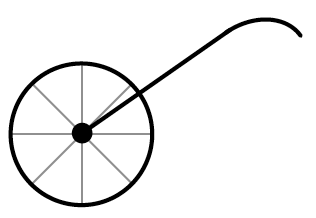### Home > PC3 > Chapter 2 > Lesson 2.1.2 > Problem2-24

2-24.A trundle wheel (a wheel attached to the end of stick) is used to measure distance. As you walk with the wheel on the ground, it makes a click after each revolution.

1. If the wheel has a radius of one foot, what is the exact distance for one revolution?

What is the circumference of the wheel?

$2\pi$(radius) = circumference

2. What would the radius need to be so that each revolution is equal to exactly $10$ feet?

Set the formula for the circumference equal to $10$. Solve for the radius.